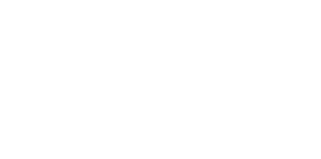## Questions1. Use trapezoidal rule to estimate the area bounded by the curve y = 8 + 2x – x2 for -1 ≤ x ≤ 3 using 5 ordinates
2.
1. Using trapezoidal rule, estimate the area under the curve y = ½x2 – 2 between x = 2 and x = 8 and x-axis. Use six strips
2.
1. Use integration to evaluate the exact area under the curve
2. Find the percentage error in calculating the area using trapezoidal rule
3. The figure below shows the graphs of y = 2x + 3 and y = -2x2+ 3x + 41. determine the co-ordinates of Q, the intersection of the two graphs
2. Find the exact area of the shaded region
4. The table below shows some values of the function; y = x2 + 2x – 3 for -6≤ x ≤ -3
 x -6 -5.75 -5.5 -5.25 -5 -4.75 -4.5 -4.25 -4 -3.75 -3.5 -3.25 -3 y 21 18.56 14.06 10.06 8.25 5 2.25 1.06 0

1.  complete the table
2. using the completed table and the mid-ordinate rule with six ordinates, estimate the area of the region bounded by the curve; y = x2 + 2x – 3 and the lines y = 0 , x = -6 and x = -3
3.
1. by integration find the actual are of the region in (b) above
2. Calculate the percentage error arising from the estimate in (b)
5. Complete the table below for y = 5x2 – 2x + 2. Estimate the area bounded by the curve, the x – axis, the lines x = 2 and x = 7 using the trapezoidal rule with strips of unit length.
 x 2 2.5 3 3.5 4 4.5 5 5.5 6 6.5 7 y 18 56.25 74 117 200.251. h = 3 - - 1 = 4 = 0.8
5         5
 x -1 -0.2 0.6 1.4 2.2 3 y 5 7.56 8.84 8.84 7.56 5
A= ½(0.8) (5 + 5 ) + 2 ( 7.56 + 8.84 + 8.84 + 7.56)
= 0.4 [10+ 2(32.8)]
= 0.4 x 75.6
= 30.24 sq. units
1. yo= 0
y1 = 2.5
y2 = 6
y3 = 10.5
y4 = 16
y5 = 22.5
y6 = 30
A= ½ x 1(0+30) + 2(2.5 + 6 + 10.5 + 16 + 22.5)
= ½ x 145= 72.5
2. ½ x2 – 2 = x3– x
6
= 83 – 8 – 23 - 2
6           6
= 77.33 - -0.67
= 78 square units
3.  % error = 72.5 – 78 x 100
78
= -7.05%
1. -2x2 + 3x + 4 = 2x + 3
-2x
2 + x +1 = 0
- 2x
2 + 2x – x + 1 = 0
(x-1) (-2x-) =0
x = 1 or x = -½
when x = 1 y = 2x 1 + 3 =5
Q (1 , 5)
2.  -2x2 + 3x + 4)dx – (2x+3)dx
1.
 X -5.5 -5 -4.25 -3.75 y 16.25 12 6.56 3.56
2. A = 0.5 (18.56 + 14.06 + 10.06 + 6.56 + 3.56 + 1.06)
= 0.5 X 53.86 = 26.93
1. x1 + 2x - 3
[x
3 + x2 – 3x]-3
3
= [ (-3)
3 + (-3)2 – 3(-3) ]
3
= 9 + 18= 27 square units
2. 27 – 26.93 X 100
27
= 0.25925 % = 0.2593 %

2.  x 2 2.5 3 3.5 4 4.5 5 5.5 6 6.5 7 y 18 28.25 4 1 56.25 7 4 94.25 1 1 7 142.25 1 7 0 200.25 233
Area{ = ½ n (y0 + yn) + 2(y1 + …………} yn - 1
= ½{(1) (18 + 233) + 2(41 + 74 + 55 + 170)}
= ½ {251 + 2(340)}
= ½ (251 + 680)
= ½ (831)
= 415.5 sq. units